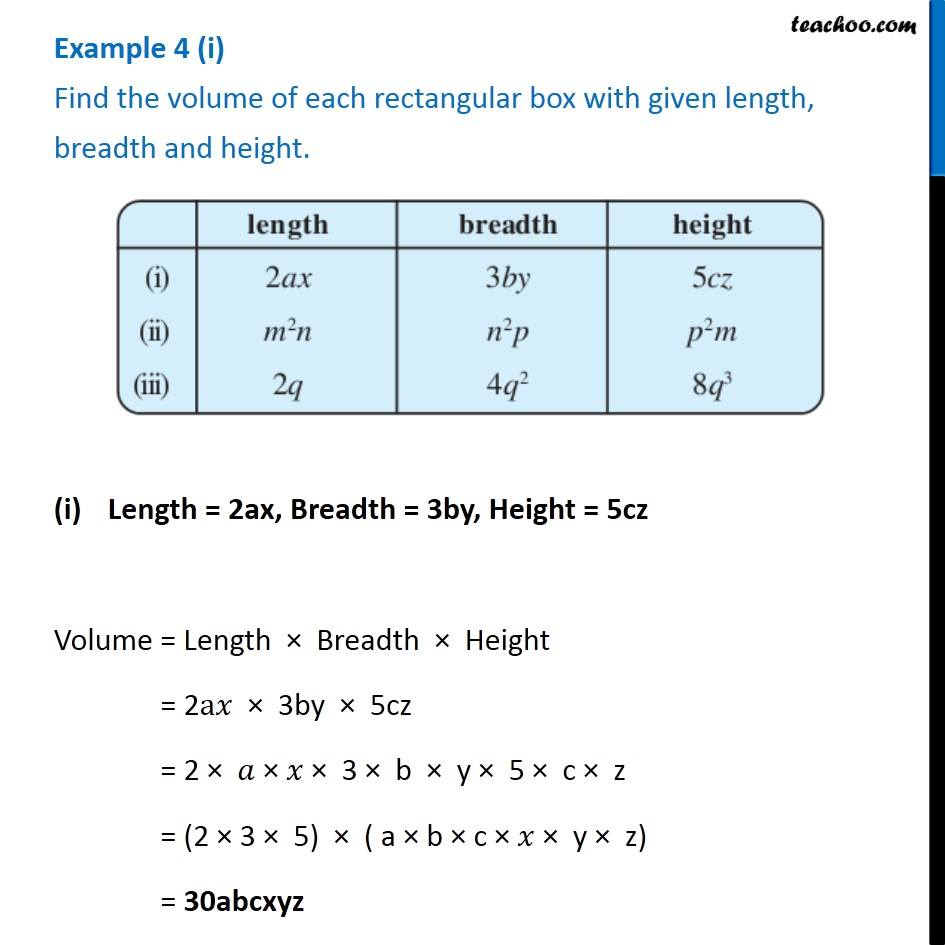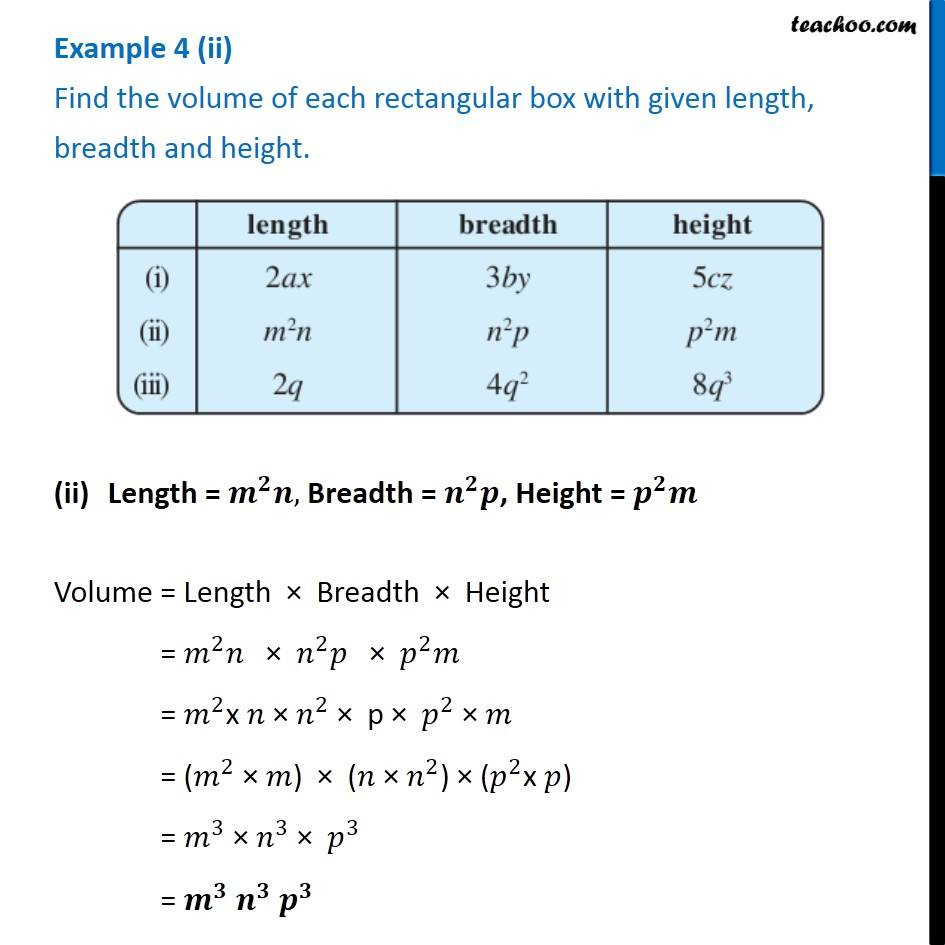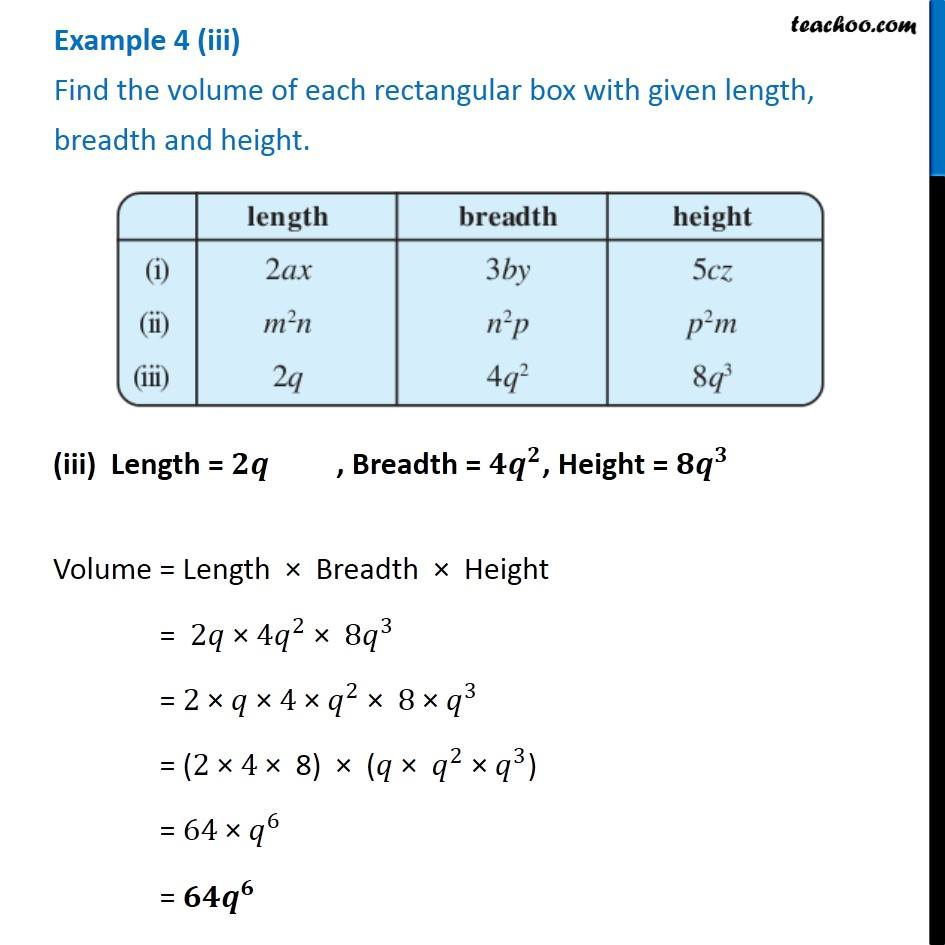Examples

Chapter 9 Class 8 Algebraic Expressions and Identities
Serial order wise### Transcript

Example 4 (i) Find the volume of each rectangular box with given length, breadth and height. Length = 2ax, Breadth = 3by, Height = 5cz Volume = Length × Breadth × Height = 2a𝑥 × 3by × 5cz = 2 × 𝑎 × 𝑥 × 3 × b × y × 5 × c × z = (2 × 3 × 5) × ( a × b × c × 𝑥 × y × z) = 30abcxyz Example 4 (ii) Find the volume of each rectangular box with given length, breadth and height. Length = 𝒎^𝟐 𝒏, Breadth = 𝒏^𝟐 𝒑, Height = 𝒑^𝟐 𝒎 Volume = Length × Breadth × Height = 𝑚^2 𝑛 × 𝑛^2 𝑝 × 𝑝^2 𝑚 = 𝑚^2x 𝑛 × 𝑛^2 × p × 𝑝^2 × 𝑚 = (𝑚^2 × 𝑚) × (𝑛 × 𝑛^2) × (𝑝^2x 𝑝) = 𝑚^3 × 𝑛^3 × 𝑝^3 = 𝒎^𝟑 𝒏^𝟑 𝒑^𝟑 Example 4 (iii) Find the volume of each rectangular box with given length, breadth and height. (iii) Length = 𝟐𝒒 , Breadth = 〖𝟒𝒒〗^𝟐, Height = 〖𝟖𝒒〗^𝟑 Volume = Length × Breadth × Height = 2𝑞 × 〖4𝑞〗^2 × 〖8𝑞〗^3 = 2 × 𝑞 × 4 × 𝑞^2 × 8 × 𝑞^3 = (2 × 4 × 8) × (𝑞 × 𝑞^2 × 𝑞^3) = 64 × 𝑞^6 = 𝟔𝟒𝒒^𝟔